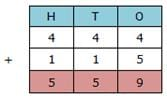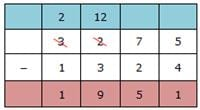Test: Roman Numerals- 1

# Test: Roman Numerals- 1

Test Description

## 10 Questions MCQ Test Mathematics for Class 5 | Test: Roman Numerals- 1

Test: Roman Numerals- 1 for Class 5 2022 is part of Mathematics for Class 5 preparation. The Test: Roman Numerals- 1 questions and answers have been prepared according to the Class 5 exam syllabus.The Test: Roman Numerals- 1 MCQs are made for Class 5 2022 Exam. Find important definitions, questions, notes, meanings, examples, exercises, MCQs and online tests for Test: Roman Numerals- 1 below.
Solutions of Test: Roman Numerals- 1 questions in English are available as part of our Mathematics for Class 5 for Class 5 & Test: Roman Numerals- 1 solutions in Hindi for Mathematics for Class 5 course. Download more important topics, notes, lectures and mock test series for Class 5 Exam by signing up for free. Attempt Test: Roman Numerals- 1 | 10 questions in 15 minutes | Mock test for Class 5 preparation | Free important questions MCQ to study Mathematics for Class 5 for Class 5 Exam | Download free PDF with solutions
 1 Crore+ students have signed up on EduRev. Have you?
Test: Roman Numerals- 1 - Question 1

### Which of the following is not a symbol to write Roman numerals?

Detailed Solution for Test: Roman Numerals- 1 - Question 1

There are 7 letters which can be used as symbols to represent Roman numerals.
They are I, V, X, L, C, D and M.
N is not used as a symbol to write Roman numerals.

Test: Roman Numerals- 1 - Question 2

### Which of the following is shown as a repetition of symbols to write Roman numerals?

Detailed Solution for Test: Roman Numerals- 1 - Question 2

Repetition of the basic symbols I, X, C and M means addition.

Test: Roman Numerals- 1 - Question 3

### Which of the following options is correct according to the rules of writing Roman numerals?

Detailed Solution for Test: Roman Numerals- 1 - Question 3
• XDVII is not written correctly as X cannot be subtracted from D. X can only be subtracted from L and C.
• CLLVV is not written correctly as V and L cannot be repeated.
• DDXC is not written correctly as D cannot be repeated.

Hence, MDCLXXI is correct according to the rules of writing Roman numerals.

Test: Roman Numerals- 1 - Question 4

Which of the following options is written in the ascending order?

Detailed Solution for Test: Roman Numerals- 1 - Question 4
• XCVII = XC + VII = 90 + 7 = 97
• CXCIV = C + XC + IV = 100 + 90 + 4 = 194
• DCCLXVIII = DCC + LX + VIII = 700 + 60 + 8 = 768
• MDCLXII = M + DC + LX + II = 1000 + 600 + 60 + 2 = 1662

So, 97 < 194 < 768 < 1662
Hence, the correct ascending order is XCVII < XCCIV < DCCLXVIII < MDCLXII.

Test: Roman Numerals- 1 - Question 5

Which of the following numerals is equal to CDXLVIV + CXV?

Detailed Solution for Test: Roman Numerals- 1 - Question 5

CDXLIV
= CD + XL + IV
= 400 + 40 + 4
CXV
= 100 + 10 + 5
= 115
Now, 444 + 115 can be solved as444 + 115 = 559
Now, 559
= 500 + 50 + 9
= D + L + IX = DLIX
Hence, CDXLIV + CXV = DLIX

Test: Roman Numerals- 1 - Question 6

Which of the following is the Hindu-Arabic form for the Romal numeral MCDXCVII?

Detailed Solution for Test: Roman Numerals- 1 - Question 6

MCDXCVII
= M + CD + XC + VII
= 1000 + 400 + 90 + 7
= 1497

Test: Roman Numerals- 1 - Question 7

Which of the following is the Roman numeral for the number 2765?

Detailed Solution for Test: Roman Numerals- 1 - Question 7

2765
= 2000 + 700 + 60 + 5
= MM + DCC + LX + V
= MMDCCLXV
Hence, MMDCCLXV is the Roman numeral for 2765.

Test: Roman Numerals- 1 - Question 8

Which of the following options is equal to MMMCCLXXV − MCCCXXIV?

Detailed Solution for Test: Roman Numerals- 1 - Question 8

MMMCCLXXV
= MMM + CC + LXX + V
= 3000 + 200 + 70 + 5
= 3275
MCCCXXIV
= M + CCC + XX + IV
= 1000 + 300 + 20 + 4
= 1324
3275 − 1324 can be solved asTherefore,
3275 − 1324 = 1951
But, 1951
= 1000 + 900 + 50 + 1
= M + CM + L + I
= MCMLI
Hence,  MMMCCLXXV − MCCCXXIV = MCMLI

Test: Roman Numerals- 1 - Question 9

Which of the following options is true?

Detailed Solution for Test: Roman Numerals- 1 - Question 9

A. XCVIII = XC + VIII = 90 + 8 = 98
MCVIII = M + C + VIII = 1000 + 100 + 8 = 1108
Which means XCVIII < MCVIII.
Hence, the statement XCVIII > MCVIII is false.
B. DCVIII = DC + VIII = 600  + 8 = 68
MCVIII = 1108
Hence, the statement DCVIII < MCVIII is true.
C. DCVIII = 68 MCVIII = 1108
Which means DCVIII < MCVIII.
Hence, the statement DCVIII = MCVIII is false.
D. XCVIII = 98
LXXXVIII = LXXX + VIII = 80 + 8 = 88
Which means XCVIII > LXXXVIII.
Hence, the statement XCVIII < LXXXVIII is false.

Test: Roman Numerals- 1 - Question 10

Which of the following symbols can be subtracted from V and X?

Detailed Solution for Test: Roman Numerals- 1 - Question 10

Only I can be subtracted from V and X.

## Mathematics for Class 5

9 videos|54 docs|35 tests
 Use Code STAYHOME200 and get INR 200 additional OFF Use Coupon Code
Information about Test: Roman Numerals- 1 Page
In this test you can find the Exam questions for Test: Roman Numerals- 1 solved & explained in the simplest way possible. Besides giving Questions and answers for Test: Roman Numerals- 1, EduRev gives you an ample number of Online tests for practice

## Mathematics for Class 5

9 videos|54 docs|35 tests